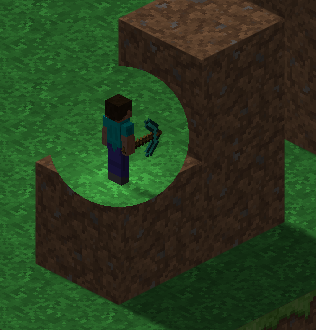I have a simple shader that basically crops a circle portion of a render texture.

But on free aspect (and on any resolution not squared) I get an ellipse for obvious reason: as I understand, texture coordinates are mapped from 0 to 1 regardless of resolution.

Is it possible to fix it somehow?

I tried to add additional POT texture and crop circle from there and then use that information to draw final pixels, but it doesn’t work.

Here’s the shader, just in case, with additional texture I mentioned above (ignore the other properties, they are leftovers from my other attempts to fix this problem):

``````Shader "Custom/CropCircle"
{
Properties
{
_MainTex("Texture", 2D) = "white" {}
_WhiteTex("White POT Texture", 2D) = "white" {}
_ScreenWidth("Screen Width", int) = 640
_ScreenHeight("Screen Height", int) = 480
}

{
Pass
{
CGPROGRAM
#pragma vertex vert
#include "UnityCG.cginc"

uniform float4 _MainTex_ST;
uniform float4 _WhiteTex_ST;

uniform sampler2D _MainTex;
uniform sampler2D _WhiteTex;

int _ScreenWidth;
int _ScreenHeight;

struct app2vert
{
float4 position: POSITION;
float2 mtc: TEXCOORD0;
float2 wtc : TEXCOORD1;
};

struct vert2frag
{
float4 position: POSITION;
float2 mtc: TEXCOORD0;
float2 wtc : TEXCOORD1;
};

vert2frag vert(app2vert input)
{
vert2frag output;
output.position = UnityObjectToClipPos(input.position);
output.mtc = TRANSFORM_TEX(input.mtc, _MainTex);
output.wtc = TRANSFORM_TEX(input.wtc, _WhiteTex);

return output;
}

fixed4 frag(vert2frag input) : COLOR
{
fixed4 main_color = tex2D(_MainTex, input.mtc);

float aspect = (float)_ScreenWidth / (_ScreenHeight == 0) ? 1.0 : (float)_ScreenHeight;

float x = input.wtc.x;
float y = input.wtc.y;

float d = sqrt(pow(0.5 - x, 2) + pow(0.5 - y, 2));

return fixed4(main_color.r, main_color.g, main_color.b, (d < _Radius) ? 1.0 : 0.0);
}
ENDCG
}
}
}
``````

Jwizard93 is right on the money:

``````float x = (0.5-input.wtc.x)*aspect;
float y = (0.5-input.wtc.y)/aspect;

float d = sqrt(pow( x, 2) + pow( y, 2));
``````

OK, as usual, the problem was a stupid one. It’s this line:

float aspect = (float)_ScreenWidth / (_ScreenHeight == 0) ? _ScreenWidth : (float)_ScreenHeight;

which should be:

float aspect = (float)_ScreenWidth /((_ScreenHeight == 0) ? _ScreenWidth : (float)_ScreenHeight);

I tried to calculate aspect in Unity and pass it as a float into shader and when I wrote the first line I got an error “cannot divide float with bool” or something. So, looks like, in a shader there was some kind of implicit conversion to avoid such error.

And you can do it on the main texture.

Stupid me.